POINT OF INTEREST 2
VALUES OF TRIGONOMETRIC RATIOS OF ANGLES OF ANY MAGNITUDELet's place a right triangle into the Rectangular Coordinate System so that the right angle lies opposite the origin.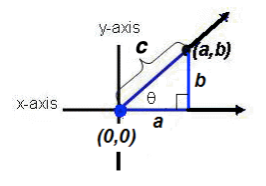Observe that the length of leg a and the length of leg b produce the point (a, b) in the coordinate system and the hypotenuse c has length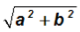(Pythagorean Theorem).

For the subsequent discussion, we will use a 30-60-90 triangle. Specifically, we will draw right triangles containing a 30o and 60o angle into each quadrant of a coordinate system. We let the 60o angle be next to the origin.

NOTE: We could have also used a 45-45-90 triangle.

Specific to our discussion, we will let the length of the side adjacent to angle 60o equal x = 1 . From geometry we know that the hypotenuse of a 30-60-90 triangle is then of length 2x = 2(1) = 2, and the other leg is of length.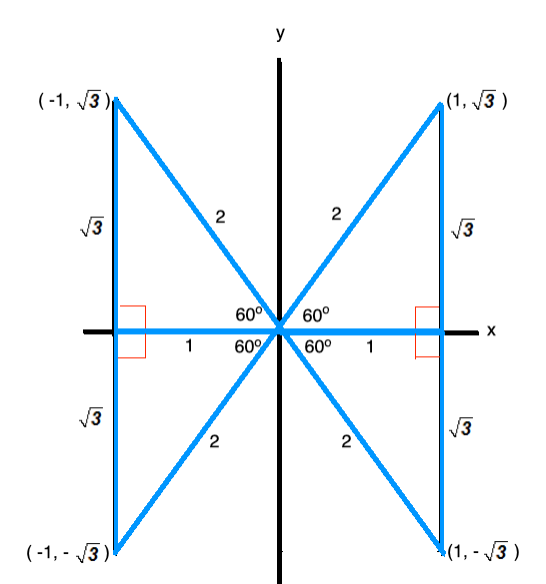As we can see, these triangles produce the points,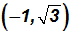,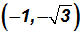, and.

Now, instead of using the sides of the triangles to define the values of the cosine, sine, and tangent ratios, we will use the four points stated above.

Their their x-coordinates will be equivalent to the sides adjacent, and their y-coordinates will be equivalent to the sides opposite the 60o angle.

Angle 60o

Its initial side is the positive x-axis, and we define its terminal side to be the line through the pointin Quadrant I.

Using adj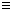1 and hyp2, we find cos 60o =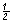.

Using opp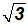and hyp2, we find sin 60o =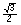.

Using oppand adj1, we find tan 60o =.

The secant, cosecant, and cotangent will all be positive.

Angle 120o

Its initial side is the positive x-axis, and we define its terminal side to be the line through the pointin Quadrant II.

Using adj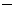1 and hyp2, we find cos 120o =.

Using oppand hyp2, we find sin 120o =.

Using oppand adj1, we find tan 120o =.

The secant and cotangent will be negative and the cosecant will be positive.

Angle 240o.

Its initial side is the positive x-axis, and we define its terminal side to be the line through the pointin Quadrant III.

Using adj1 and hyp2, we find cos 240o =.

Using oppand hyp2, we find sin 240o =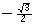.

Using oppand adj1, we find tan 240o =.

The secant and cosecant will be negative and the cotangent will be positive.

Angle 300o.

Its initial side is the positive x-axis, and we define its terminal side to be the line through the pointin Quadrant IV.

Using adj1 and hyp2, we find cos 300o =.

Using oppand hyp2, we find sin 300o =.

Using oppand adj1, we find tan 300o =.

The cosecant and cotangent will be negative and the secant will be positive.

Do you notice in the above that some values are negative? As a matter of fact, we can actually establish the following pattern for any angle.Do you further notice in the above that the multiples of angle 60o produce values equal to the absolute value of the values in QI? This finding allows us to understand how some values of trigonometric ratios are connected.